## Rectifier Control of DC Series Motor:

Single-phase controlled Rectifier Control of DC Series Motor are employed in traction. A single-phase half-controlled Rectifier Control of DC Series Motor is shown in Fig. 5.37(a). Equivalent circuit of motor is also shown. Since back emf decreases with armature current, discontinuous conduction occurs only in a narrow range of operation. Hence, it will be neglected here. The waveforms of va,ia and instantaneous back emf e for continuous conduction are shown in Fig. 5.37(b).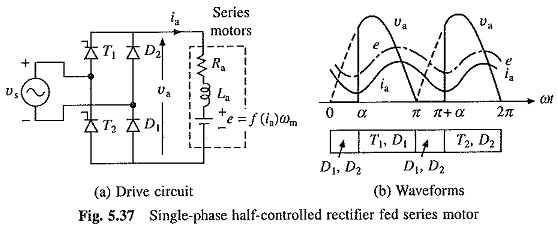Although, in steady state, fluctuations in speed are negligible, e is not constant but fluctuates with ia. For a given speed, e is related to ia through magnetization curve of motor, which is nonlinear owing to saturation. Thus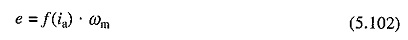Motor operation is described by following equations for duty and freewheeling intervals respectively,Because of the presence of term f(ia), Eqs. (5.103) and (5.104) are nonlinear differential equations and can only be solved numerically. A simple method of analysis is obtained when e is replaced by its average value Ea such thatwhere

Since the drop across the inductance La due to dc component of armature current Ia is zero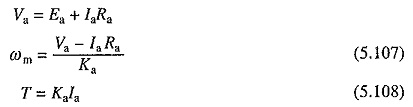For continuous conduction, Va for half-controlled and fully-controlled single-phase rectifiers is given by Eqs. (5.93) and (5.83), respectively.

Following sequence of steps are used to calculate speed-torque characteristic for a given α taking into account non-linearly of the magnetic circuit: A value is chosen for Ia. Corresponding value of Ka is obtained from the magnetization characteristic of the motor. For the known value of α, calculate Va from Eq. (5.93) or (5.83), depending on the rectifier circuit used. Now ωm and T are obtained from Eqs. (5.107) and (5.108), respectively. Nature of speed-torque characteristics for the drive of Fig. 5.37(a) is shown in Fig. 5.38.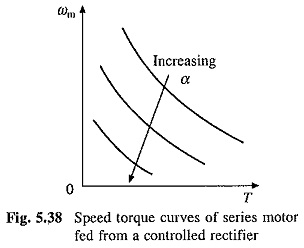### Control of Fractional hp Motors: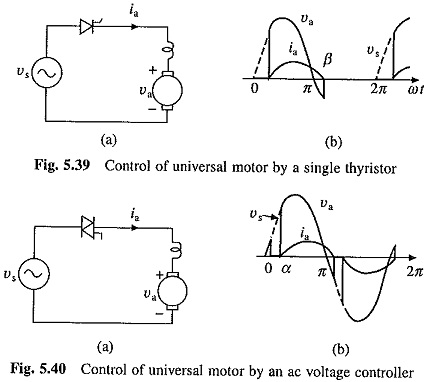Because of low cost single-phase half-wave controlled rectifier of Fig. 5.39(a), employing a single thyristor, is commonly used for the control of fractional hp universal, dc series and permanent-magnet dc motors. Such drives are employed in hand tools and small domestic appliances.

Motor terminal voltage and armature current waveforms for universal motor are shown in Fig. 5.39(b). The drive operates is discontinuous conduction with a large zero current interval and large current ripple. Consequently, efficiency is poor, speed regulation is large and speed may fluctuate around its average value when the inertia is low. Sometimes a freewheeling diode is added to reduce the duration of zero current interval.

Universal motors may also be controlled by a triac ac voltage controller as shown in Fig. 5.40(a). The triac is fired at α and (π + α). Now the machine armature carries ac current (Fig. 5.40(b)). Because of reduced duration of zero current interval, the drive has negligible speed fluctuations and lower speed regulation.

Scroll to Top